Courses

# Test: Free Energy Change And Spontaneity

## 16 Questions MCQ Test Chemistry for JEE | Test: Free Energy Change And Spontaneity

Description
This mock test of Test: Free Energy Change And Spontaneity for JEE helps you for every JEE entrance exam. This contains 16 Multiple Choice Questions for JEE Test: Free Energy Change And Spontaneity (mcq) to study with solutions a complete question bank. The solved questions answers in this Test: Free Energy Change And Spontaneity quiz give you a good mix of easy questions and tough questions. JEE students definitely take this Test: Free Energy Change And Spontaneity exercise for a better result in the exam. You can find other Test: Free Energy Change And Spontaneity extra questions, long questions & short questions for JEE on EduRev as well by searching above.
QUESTION: 1

### Direction (Q. Nos. 1- 8) This section contains 8 multiple choice questions. Each question has four choices (a), (b), (c) and (d), out of which ONLY ONE option is correct. Q. For the given reaction,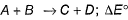= - 1.3818 kcal at 300 K. Thus equilibrium constant is

Solution:

Since the reaction is not reversible, equilibrium constant is zero.

QUESTION: 2

### ΔHvap = 30 kJ mol-1 and ΔSvap = 75 J mol-1 K-1. Thus, temperature of vapour at one atmosphere is [IIT JEE 2004]

Solution:

∆HVap = 30kJ/mol and ∆S = 75 J/K
∆G = ∆H - T∆S
At eqm, ∆G = 0
Therefore, ∆H = T∆S
Or T = 30×103/75 = 400 K

QUESTION: 3

### Which reaction, with the following values of ΔH and ΔS at 400 K is spontaneous and endothermic?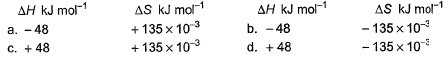Solution:

∆G = ∆H - T∆S
For opt (c), ∆G = 48000 - 400(135)
= 48000 - 54000
= -6000
∆G is -ve
Therefore reaction is spontaneous.

QUESTION: 4

Standard entropies of X2, Y2 and XY3 are given below the reaction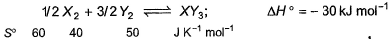Q. At what temperature, reaction would be in equilibrium?

Solution:

1/2X + 3/2Y2 ⟶XY3,
ΔH= −30 kJ
ΔSreaction = ∑ΔSproduct−∑ΔSreactant
X+ 3Y→ 2XY3
​ΔH=−60 kJ
ΔSreaction = 2×50−3×40−1×60 =100−120−60=−80 JK−1mol−1
ΔG=ΔH−TΔS=0
ΔH=TΔS
1000×(−60)=−80×T
T=750 K

QUESTION: 5

The value of log10 K for a reaction, A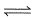B is (Given, ΔH°298 = - 54.07 kJ mol-1;

ΔS°298 = + 10 JK-1 mol-1; R = 8.314 JK-1 mol-1 2.303 x 8.314 x 298 = 5705)

[IITJEE2007]

Solution:

∆G° = ∆H° - T∆S°
= -54070 - 298 10
= -57050
∆G° = -2.303 RT log10k
-57050 = -2.303 8.314 298 log10k
57050 = 5705 log10k
log10k = 10

QUESTION: 6

For the following decom position reaction,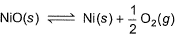ΔG° = 1729 J mol-1 at 300 K , (log 5 = 0.6990]

Thus, pressure set up is

Solution:
QUESTION: 7

If for the cell, Zn(s) + Cu2+(ag)Cu(s) + Zn2+ (ag)entropy change ΔS° is 96.5 JK-1 mol-1, then temperature coefficient of the emf of a cell is

Solution:

ΔG=ΔH−nFT(dE/dT)P
​and  ΔG=ΔH−TΔS
∴ΔS/nF=(dE/dT)P
or
96.5/2×96500=(dE/dT)P
​∴(dEcell/dT)P
​=1×10−3 / 2
=5×10−4VK−1

QUESTION: 8

For the reaction, at 1000° C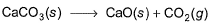ΔG° = - 27 kJ mol-1,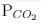= 0.0033 atm.

Q. Hence, ΔG at this temperature is

Solution:
QUESTION: 9

Direction (Q. No. 9) This sectionis based on statement I and Statement II. Select the correct answer from the code given below.

Q.

Statement I :Every endothermic reaction is spontaneous if TΔS > ΔH.

Statement II : Sign of ΔG is the true criterion for deciding spontaneity of a reaction.

Solution:
*Multiple options can be correct
QUESTION: 10

Direction (Q. Nos. 10 and 11) This section contains 2 multiple choice questions. Each question has four choices (a), (b), (c) and (d), out of which ONE or  MORE THANT ONE  is correct.

Q. Which of the following statements is/are true?

Solution:

The entropy of a substance increases on going from the liquid to the vapour state at any temperature. It is true. As randomness increases, entropy also increases.
∆G = ∆H - T∆S. So for spontaneous reaction, all 3(T, ∆H and ∆S) are needed.
An exothermic reaction will always be spontaneous. THis is false
Reactions with a positive (ΔH° and ΔS°) can never be product favoured. False, for a larger value of T, ∆G might be negative.
If ΔG° for a reaction is negative, the reaction will have an equilibrium constant greater than one. True, for ΔG° less than 0, reaction is spontaneous and so, ith=s eqm constant will have value greater than 1.

*Multiple options can be correct
QUESTION: 11

When HCI(g)and NH3(g)come in contact, they react producing a white cloud of solid NH4CI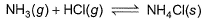For this,

Solution:

According to me, correct options are b and d.
For a reversible reaction, ∆S°(Universe) will be zero. ∆S°(Universe) is greater than zero for an irreversible reaction. SInce the randomness decreases, ∆S°(system) becomes less than zero and ∆S°(surrounding) becomes more than zero. BOth becomes equal with sign opposite and thus for reversible reaction, ∆S°(universe) = ∆S°(System) + ∆S°(surrounding) = 0

QUESTION: 12

Direction (Q. Nos. 11-14) This section contains 2 paragraph, wach describing theory, experiments, data etc. three Questions related to paragraph have been given.Each question have only one correct answer among the four given options (a),(b),(c),(d).

Passage l
Sulphur undergoes a phase transition between 80 and 110°C
S(rhombic)S (monoclinic); ΔH° = 3.213 kJ mol-1; ΔS° = 8.71 JK-1 mol-1
Q. Select the correct alternate(s).

Solution:

We have ∆G = ∆H - T∆S
For spontaneous reaction ∆G<0 and vice versa,
∆G at 80° C:-
3213 - (80+273)8.73 = 141.9 kJ mol-1
As it comes positive, the conversion of rhombic and monoclinic is non- spontaneous at 80° C.In other words, rhombic is more stable than monoclinic at this temperature.
∆G at 110° C:-
3213 - (110+273)8.73 = -119.1 kJ mol-1
As it comes positive, the conversion of rhombic and monoclinic is spontaneous at 110° C.In other words, rhombic is less stable than monoclinic at this temperature.

QUESTION: 13

Passage l

Sulphur undergoes a phase transition between 80 and 110°C
S(rhombic)S (monoclinic); ΔH° = 3.213 kJ mol-1; ΔS° = 8.71 JK-1 mol-1
Q. Temperature at which ΔG° = 0, is

Solution:

We have ∆G = ∆H - T∆S
At eqm. ∆G=0,
T = ∆H/∆S
= 3.213 kJ mol-1 / 8.71 JK-1 mol-1
= 368.88 K

QUESTION: 14

Passage II

For oxidation of iron at 298 K, 4 Fe (s) + 3 O2(g) → 2 Fe2O3(s)
ΔS° = - 549.4 JK-1 mol-1 and ΔH ° = - 1648 . 0 kJ mol-1

Q. Select the correct alternate.

Solution:
QUESTION: 15

Passage II

For oxidation of iron at 298 K, 4 Fe (s) + 3 O2(g) → 2 Fe2O3(s)
ΔS° = - 549.4 JK-1 mol-1 and ΔH ° = - 1648 . 0 kJ mol-1

Q. Free energy change for this reaction is

Solution:

∆G = ∆H - T∆S
= -1648000 - 298 × (-549.4)
= -1648000 + 163721.2
= - 1484278.8
= - 1484.28kJ

QUESTION: 16

Direction (Q. Nos. 16) Choice the correct combination of elements and column I and coloumn II  are given as option (a), (b), (c) and (d), out of which ONE option is correct.

Q. Thermodynamic equilibrium constant K is related to temperature by equation log10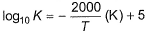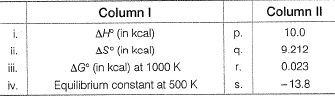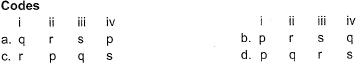Solution: